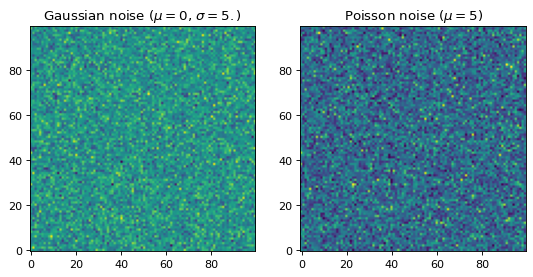# make_noise_image¶

photutils.datasets.make_noise_image(shape, distribution='gaussian', mean=None, stddev=None, seed=None)[source]

Make a noise image containing Gaussian or Poisson noise.

Parameters
shape2-tuple of int

The shape of the output 2D image.

distribution{‘gaussian’, ‘poisson’}

The distribution used to generate the random noise:

• 'gaussian': Gaussian distributed noise.

• 'poisson': Poisson distributed noise.

meanfloat

The mean of the random distribution. Required for both Gaussian and Poisson noise. The default is 0.

stddevfloat, optional

The standard deviation of the Gaussian noise to add to the output image. Required for Gaussian noise and ignored for Poisson noise (the variance of the Poisson distribution is equal to its mean).

seedint, optional

A seed to initialize the numpy.random.BitGenerator. If None, then fresh, unpredictable entropy will be pulled from the OS.

Returns
image

Image containing random noise.

Examples

# make Gaussian and Poisson noise images
from photutils.datasets import make_noise_image
shape = (100, 100)
image1 = make_noise_image(shape, distribution='gaussian', mean=0.,
stddev=5.)
image2 = make_noise_image(shape, distribution='poisson', mean=5.)

# plot the images
import matplotlib.pyplot as plt
fig, (ax1, ax2) = plt.subplots(1, 2, figsize=(8, 4))
ax1.imshow(image1, origin='lower', interpolation='nearest')
ax1.set_title('Gaussian noise ($\mu=0$, $\sigma=5.$)')
ax2.imshow(image2, origin='lower', interpolation='nearest')
ax2.set_title('Poisson noise ($\mu=5$)')